mdbtxt1
mdbtxt2
Proceed to Safety

Robert P. Munafo, 2023 Jun 14.

The escape radius is a parameter used in iterating a point under the Mandelbrot Set and Julia Set functions. It is the radius of a circle on the Complex Plane used as a boundary to determine when iteration can stop. The circle is centered at the origin, and has a radius of at least 2.0. As soon as the iteration yields a value that falls outside the circle, iteration can stop and it has been determined that the point that was iterated is not a member of the Mandelbrot Set.

A common optimization is to compare the square of an iterate's magnitude to the square of the escape radius; this is to avoid the square root calculation that would be required to compute the actual magnitude. The square of the magnitude is simply the sum of the squares of the two components, and these are computed as imtermediate results of the iteration calculation.

### Minimum Escape Radius of 2

The reason for having an escape radius is to prevent iteration from going on forever. The Mandelbrot iteration is defined to terminate when the iteration becomes unbounded, i.e. goes off to infinity. It is fairly straightforward to show that if a point ends up outside a radius of 2.0 then the iteration will go off to infinity. Therefore any escape radius greater than 2.0 will also suffice.

This proof is from Mike Hurley, posted to USENET on 1993 Apr 26:

The sequence generated by f(z) = z^2 + c beginning with 0 begins    0, c, c^2 + c, ...    Call these terms z0, z1, z2, ... .    In fact this sequence will be unbounded if any of its terms has magnitude larger than 2.    The main idea is that if the magnitude |z| is bigger than both 2 and the magnitude |c|, then |f(z)|/|z| > |z| - 1 > 1, so that by induction the sequence of magnitudes will grow geometrically.    To establish the inequality, note that    |f(z)|/|z| = |z^2 + c|/|z| >= (|z|^2-|c|)/|z| [ triangle inequality ] = |z| - (|c|/|z|) > |z| - 1 [ |z| > |c| ] > 1 [ |z| > 2 ]    If |c| <= 2 and |zn| > 2 then certainly z = zn satisfies these hypotheses, so the sequence is unbounded.    If |c| > 2 then |c^2+c| >= |c|^2 - |c| = |c|(|c|-1) > |c| > 2 so that z=z2 satisfies the hypotheses of the argument above.

The last paragraph shows that any starting point with magnitude greater than 2 will have iterates that diverge to infinity; the paragraph before that shows that any iteration that eventually contains a point with magnitude greater than 2 will likewise diverge to infinity. See the trialgle inequality article for an explanation of the first step in the derivation..

### Effects of Larger Radius Values

Using escape radius values larger than 2 provides several benefits:

Here are some elephant valley views showing the effects of different escape radius values, starting at the minimum of 2 and going up. The biggest noticable difference is in the shape of the "tiles" seen in binary decomposition. In addition, the colours change because using larger values makes it take more iterations to escape.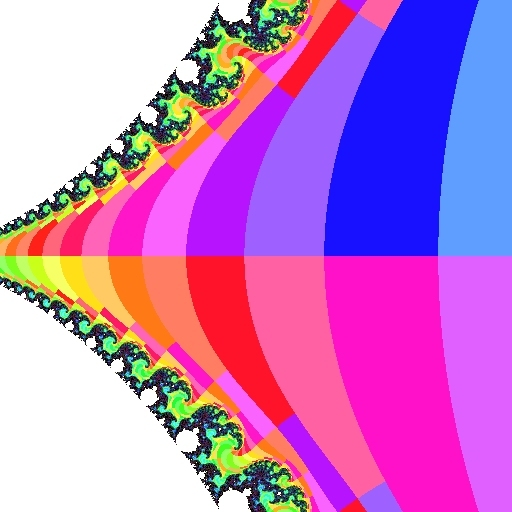escape radius 2.00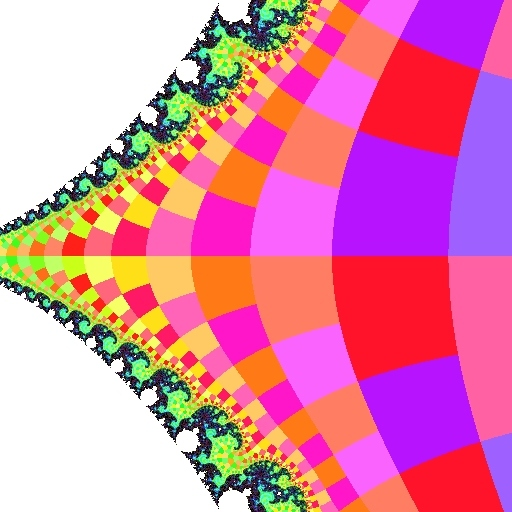escape radius 23.14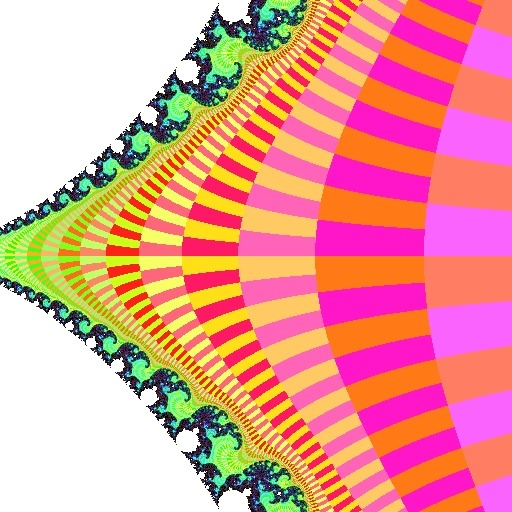escape radius 65,536

The value eπ=23.14069... is special because the "tiles" are most nearly perfect squares. A mathematical proof of this reduces to the fact that the limit (for large n) of (1+(π/n))n is eπ.

### Uneven Dwell Bands and Unaligned Tiles

With the lowest viable escape radius values (such as 2, used here) these effects are most noticable in the most west parts of the Mandelbrot set: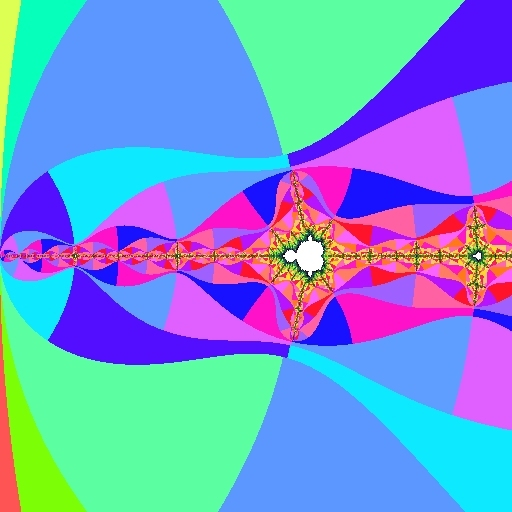Dwell bands of uneven widthUnaligned binary decomposition "tile" edges

On the left we see that the dwell bands vary in width and adjacent lemniscates touch at the west tip. On the right, the external ray line coming in from the top (its angle is 7/16) is disjointed, shifting every time it crosses a lemniscate.

### Non-Circular Escape Boundary

In early programs as an optimization the "escape" test was simply to compare the real and imaginary components of each iterate to detect if either was outside the range [-2..2]. Such a test correctly catches all non-member points, and is faster than squaring and adding. This was most useful when using integer math because the tests can be done by masking out the high bits of a fixed-point representation.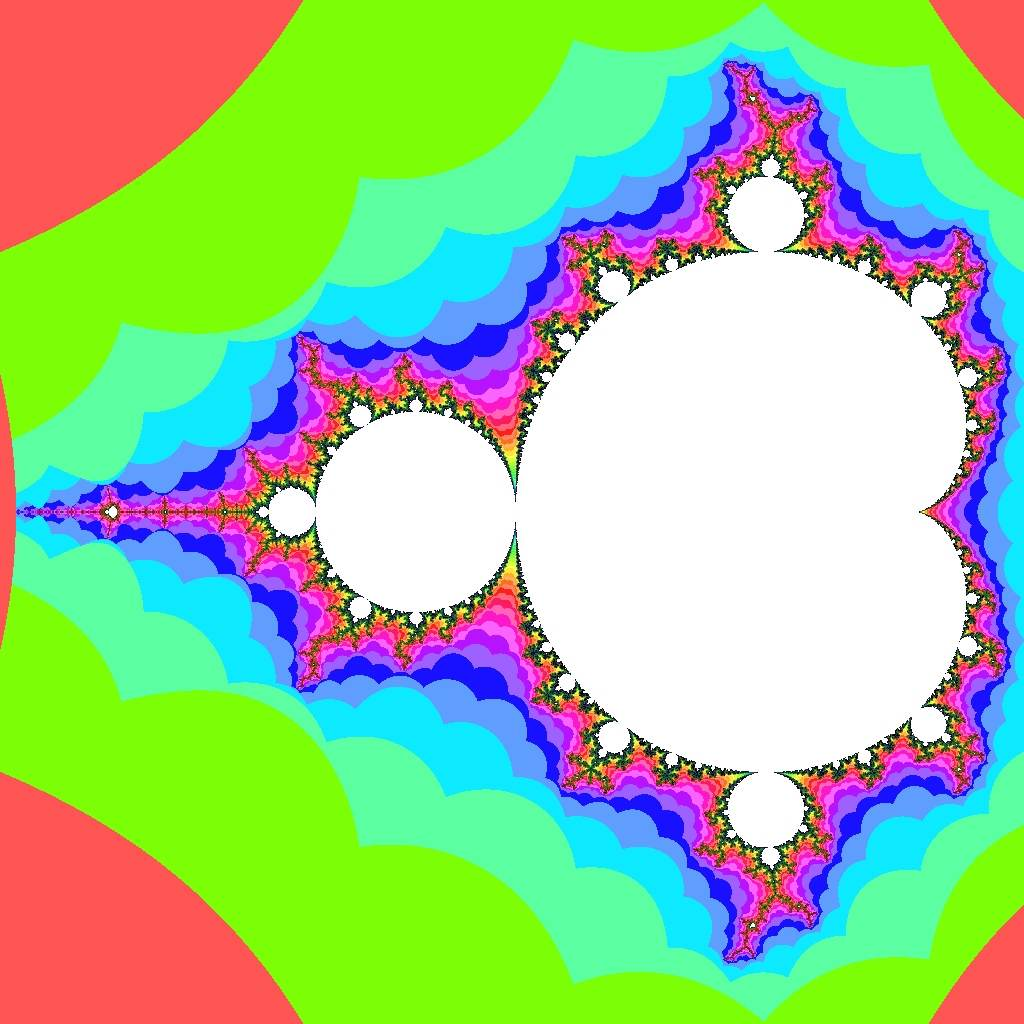Using a square escape region instead of a circle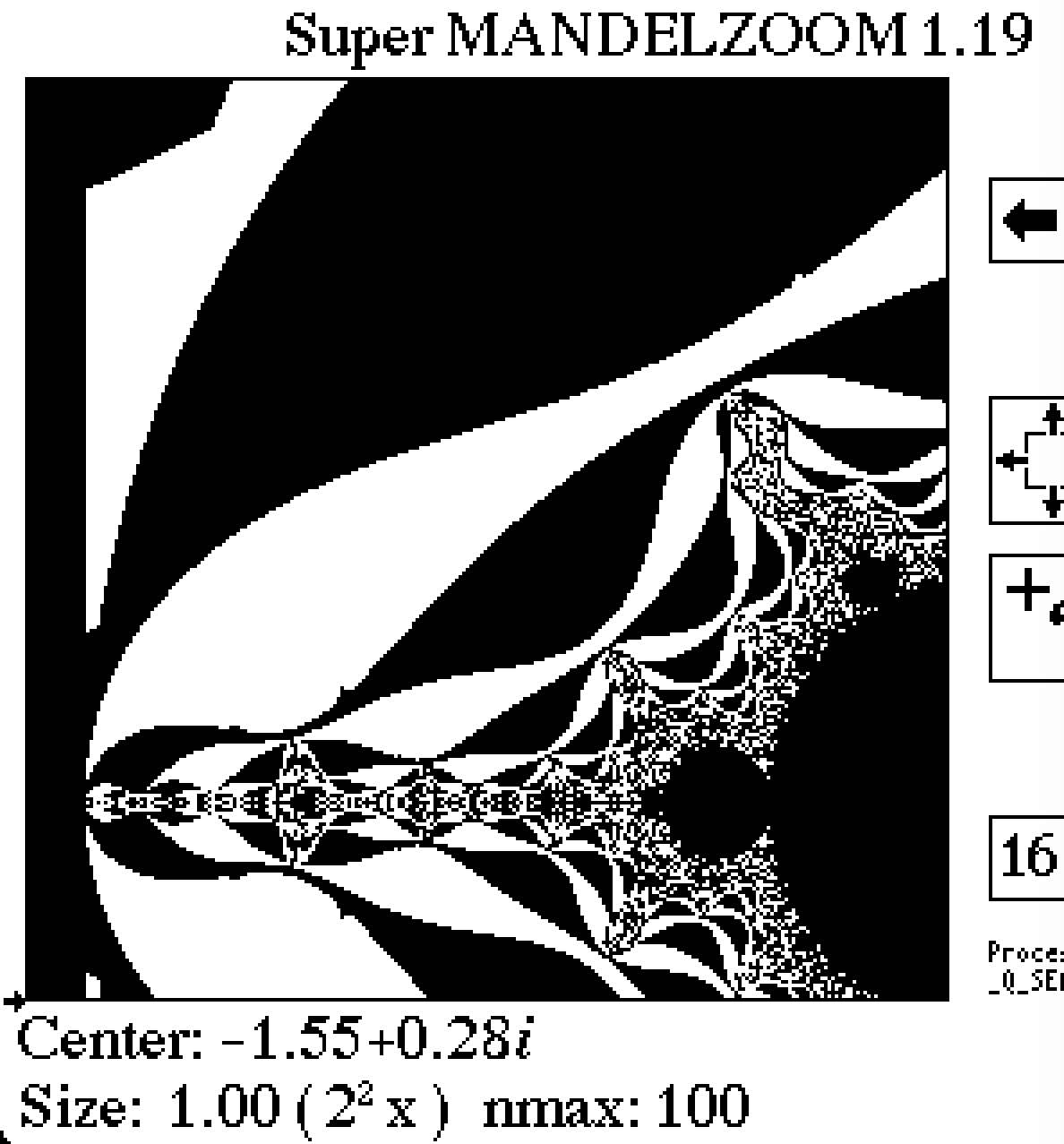Dwell band flaws in Super MANDELZOOM

Seen on the right is my early program Super MANDELZOOM. It uses this optimization, but also tests if the penultimate (not-yet-escaped) iterate is outside a circle of radius 2, adding one to the dwell in that case; this results in nearly smooth lemniscates but has visible flaws particularly in west areas of the Mandelbrot set.

Acknowledgments

Proof that 2.0 is sufficient: Mike Hurley (mgh3@po.cwru.edu)

revisions: 19971119 oldest on record; 20230320 describe optimisation; 20230614 illustrate effects of different escape radius values; 20230618 add a bit more description of illustrated flaws

From the Mandelbrot Set Glossary and Encyclopedia, by Robert Munafo, (c) 1987-2023.

This page was written in the "embarrassingly readable" markup language RHTF, and was last updated on 2023 Jun 19.s.27